# An Introduction to Quantum Computing without the Sci-Fi: From the Qubit to Quantum Teleportation

Quantum Computing is a new field that utilizes the unique properties of objects at a quantum, or very small, level. These properties allow it to be exponentially faster than classical computer at certain types of problems, including factoring (hence, buzz about quantum computing breaking RSA encryption), database searches, as well as simulating molecules, quantum states, and other complex systems. While quantum computing experts don’t think quantum computers will assist classical computers, they estimate that both types of computers can work together to solve many of Earth’s leading issues.

Yet quantum computing is so often dwarfed in heaps of science fiction and explanations that add more haze than clear it up. As we discover more about the quantum universe, there is really no way human minds can intuitively understand the physical aspect of quantum physics. When we can’t understand the world, we try to model it with mathematics.

Let’s try to understand quantum computing — and a bit of quantum physics — from a computer science and math-based perspective. While it won’t explain exactly what is going on in the physics of the quantum world, you will be able to intuitively explain representations of unintuitive and confusing phenomena, like quantum entanglement, that even Einstein scratched his head over.

Let’s get started!

# The Qubit

Let’s start at the bit. Classical computers, the widespread computer you may be reading this article on, run on bits. Bits can either be 0 or 1, and everything that is on your computer is stored in the form of bits. Physically, bits are represented by electrons passing through transistors. Using logic gates like AND or NOT, one can create diverse functions with bits.

Mathematically, we represent a bit (or many bits) in what is called a state vector. A state vector is a vector that contains all the possible values the bit or bit system can contain, and if the system is in that state or not.

We can apply some logic gates to bits. For single bits, there are only two gates: the IDEN (Identity) gate, which outputs the state that was entered (0 to 0, 1 to 1) and the NOT gate, which outputs the opposite of the state that was entered (0 to 1, 1 to 0). We can represent applying these gates as multiplying the state vector by the gate matrix.

You may notice that the identity gate is simply an identity matrix!

Now, let’s test out some gates on bits.

I will introduce a rule — the sum of the squares of each element of the state vector must equal 1. You will see why later (if this reminds you of the equation for a circle, you’re on the right path).

In the classical world, you can only have two values for a bit — 0 and 1. But in the quantum world, you can have quantum bits — qubits — that are simultaneously 0 and 1.

Think of states 0 and 1 as two pieces of music, the same volume, instead of mutually exclusive states. We have a rule where we can play one piece of music, or both at the same time, but we must keep the volume to the same volume as one piece of music. Then, it seems obvious that you could play, say, music 0 at 75% volume and music 1 at 25% volume, or some other combination, as long as the percentage equals 100%.

A quantum particle that is in a state of both 0 and 1 is in superposition. When we measure a quantum particle, we force it into either a 0 or a 1, with the probability and , respectively, where a and b are defined in the state vector for a bit: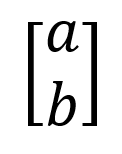State vector, where a² is the probability that the state exists in 0, and b² is the probability that the state exists in 1.

When you measure the state of a qubit, it will never be a decimal like 0.5 or 0.25; it will always be 0 or 1. The superposition only determines the probability that it will collapse into a 0 or a 1. A state like

Would measure to 0 exactly half the time and 1 the other 50% of times, because a² = one-half and b² = one-half.

the state would measure to 0 three-quarters of the time and 1 one-quarter of the time, because a² equals three-quarters and b² = one-quarter.

Test yourself — how many times, estimated out of 100 measurements, would the state vector below evaluate to 0?

It would measure to 0 an estimated 12 or 13 times (12.5, if you are using expected value) out of 100 measurements. By squaring a, we get one-eighth, which multiplied by 100 is 12.5.

From one measurement we do not know the state of a randomly given qubit — we must measure the qubit several times and see how many times it evaluates to 0 and how many times it evaluates to 1. Then, by square-rooting the probabilities for both states, we obtain the state vector.

# The Bloch Sphere and other Single-Qubit Gates

Below is the Bloch sphere, a tool to visualize the state vector of a qubit.

The Bloch sphere is a sphere of radius 1, and each state is represented by a radius from the origin to a location on the surface of the sphere. When the radius is pointing straight up, it represents the state 0. When the radius is pointing straight down, it represents the state 1.

If you’re interested in the mathematics behind the Bloch sphere, you can check out the trigonometry-heavy explanation behind it here. (It is one of many interesting uses of Euler’s Identity).

Put simply, two of the axes represent values of the state vector. (See if you can spot why the equation of a circle, x²+y²=1, dictates they must add to 1.) Because a state vector value could be imaginary/complex, these are accounted for by adding another dimension. A more satisfying answer is provided in the link above.(Note — this means that when an imaginary state-vector value is squared, the probability will be negative. This is yet another interesting element of quantum computing — negative probabilities — that is used in Grover’s Algorithm, a method to find an entry in a database with exponentially less queries than with a classical algorithm.)

You can visualize applying gates to qubits as rotations around the Bloch sphere. An X gate (quantum NOT gate) rotates a radius a vertical half-full rotation around the sphere — and this can apply not only to classical states, but also states in superposition.

Interesting! It appears that multiplying an equal superposition state by an X gate results in the same state. But how can it be? They are not in the same place on the Bloch sphere.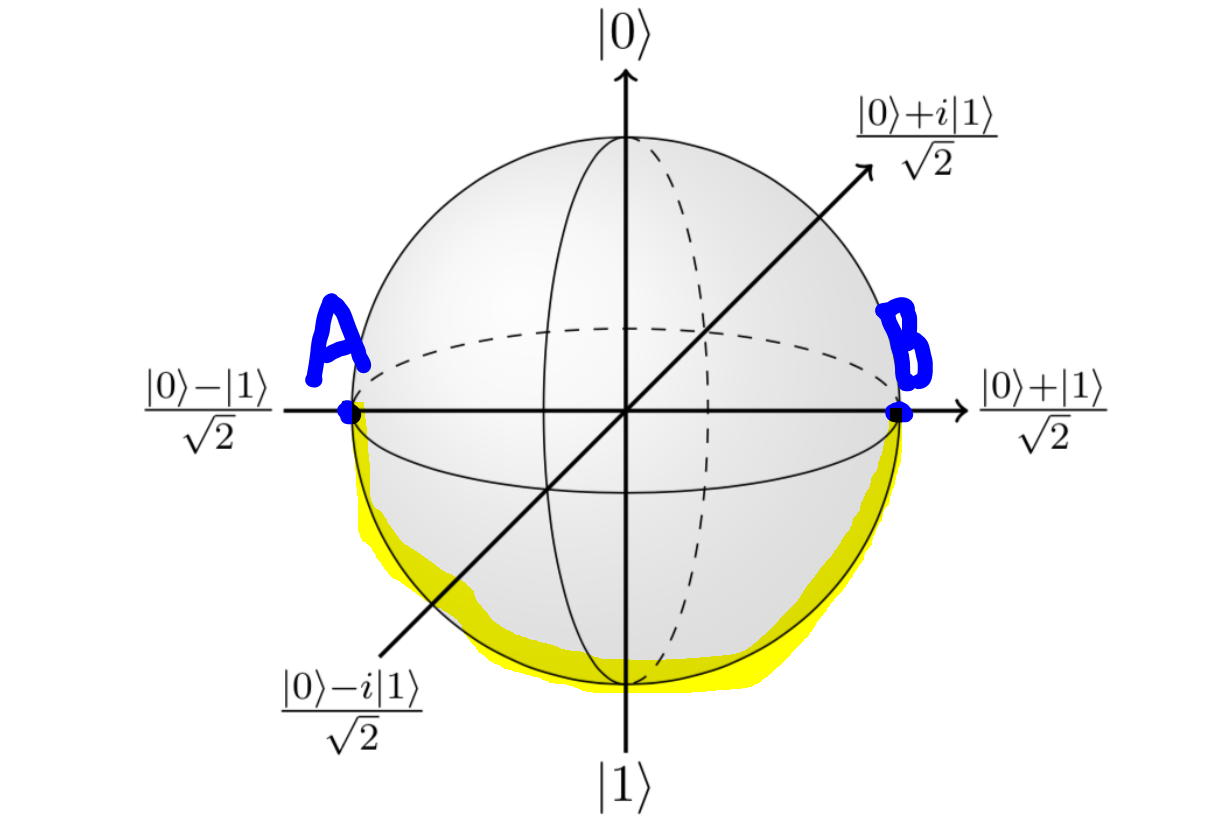The superposition, started from an arbitrary point A and made a half-full rotation vertically until it reached point B. Image: IBM (with markings)

While at different locations, point A and B are both the same distance from the poles 0 and 1. They still have the same probability of measuring to be a 0 or a 1 — in fact, this is same for every point in the mid-circle. This means that many different locations (results of applying operations) can have the same state vector.

You’ve seen how one can model transformations of qubit states using the Bloch sphere with an X gate.

Let’s introduce a few more: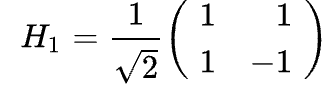The Hadamard Gate. Sub-1 denotes it is applied to only 1 qubit. There are other matrices that can be applied to 2, 3, +… qubit systems. We’ll learn about those later!

The Hadamard, perhaps the most important gate, puts deterministic states (like 0 or 1) into probabilistic states (like equal superposition).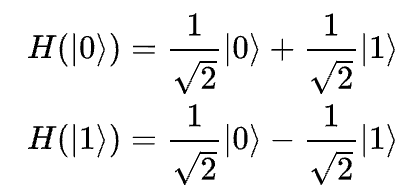The effect of the Hadamard gate on states 0 (denoted |0>) and 1 (denoted |1>). These equations are written in Dirac Notation, which is not introduced in this article.

As seen above, the Hadamard gate puts the 0 and 1 states into superposition. As we saw from the labelled Bloch Sphere above, they are the same thing in terms of state vectors, because they both measure 0 half the time and 1 the other half of the time.

The above equations show how the Hadamard gate transforms states in superposition to deterministic states.

Note — gates can be applied on any state vector, not just ones in equal superposition or deterministic states. Although the result may not be as clean, gates can be applied to any state and apply a fixed rotation.

Now, we will introduce the Y and Z gates. They, along with the X gate, are in a family called the Pauli Gates, and perform rotations along axes.

The above table also shows how gates are visualized in circuit diagrams. You may notice the presence of the imaginary unit i in the Pauli-Y gate. This has to do with the additional imaginary dimension added to the Bloch Sphere.

Some other single-qubit gates include the S/P and T gates, which perform other rotations.

In this section, you have learned how matrices and the Bloch Sphere can be used to model transformations on qubit states. You learned about how qubits can be brought into and out of superposition.

Exercise: Try starting with the state 0 and multiplying your way around to state 1 and back, without using the X gate. This will further your understand of how the Bloch Sphere and state vectors interact, which is an important property of quantum computing that is exploited by many algorithms.

Onwards to multi-qubit systems!

# Multi-Qubit Systems — the Tensor Product

Just like bits, qubits can exist in multi-bit/qubit systems. These systems have state vectors as well, which can be found by the tensor product, which looks like a circle with an x inside it.

Let’s look at the state vector for a 2-qubit system.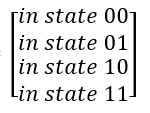Like a single-qubit state vector, each element represents the probability for a certain combination of qubits.

The below equation tensors two qubits in the state 0.

The resulting state vector is 100% in state 00, which are the two qubits that we tensored (0 and 0)!

Exercise: try tensoring the other combinations of deterministic qubit states (0 by 1, 1 by 0, 1 by 1). Then, try tensoring qubit states in various superpositions.

# Entanglement

You may have heard of entanglement — that is, when you entangle two qubits, by measuring one, the other qubit instantly adjusts to copy the other qubit’s state, even if they are separated by thousands of light years, as if there was some sort of ultra-fast communication channel. Because this phenomenon suggested that information could be transmitted faster than the speed of light, violating Einstein’s Theory of Special Relativity, it puzzled Einstein and other great physicists so much that it caused a fundamental split in the way the world was viewed.

“Spooky action at a distance,” it was described by Einstein. There are many theories as to how entanglement occurs in alignment with Einstein’s view of the universe, such as the two qubits ‘agreeing’ on the answer beforehand.

If you’re curious about the physical nature of entanglement, here is an article about entanglement occurring in drumheads in human hair from 2018.

If it puzzled Einstein, the physical aspect of entanglement probably puzzles you too. But that is the purpose of this article — to represent these physically unintuitive ideas using logical operations.

The state vector for a two-qubit entangled state is

Can you figure out why?

This state vector will output 00 half the time, and 11 half the time. From this perspective, it seems obvious how the state is ‘entangled’. If the first qubit is 0, then the other qubit must be 0. If the first qubit is 1, then the other qubit must be 1.

Another fascinating aspect of the entangled state vector is how it is de-tensored.

If you remember from the first introduction to the tensor product, each element of the state vector is simply a product of elements of the two tensored qubits.

You will realize that there are no solutions for a, b, c, and d!

You can see that there is an inherent contradiction in the equations. Therefore, that state vector cannot be separated into two independent qubits —rephrased, there is no way for the state to be written as a tensor product of two individual bits. They are entangled.

You will learn how to create entanglement in the next section!

# Multi-Qubit Gates

There are more gates that can be applied to two-qubit systems. These are the CNOT (Controlled NOT) gate, the CZ (Controlled Z) gate, and the SWAP gate.

The CNOT gate applied a NOT gate to the second qubit if the first qubit is equal to 1. Note that because the first qubit may be in superposition, a ‘fraction’ of the NOT gate can be applied to the second qubit. This concept can be explored more by applying the CNOT matrix on state vectors in superposition.

The CZ gate, like the CNOT gate, applies a Z gate to the second qubit if the first qubit is equal to 1.

The SWAP gate swaps the values of the two qubits that are entered.

Starting from two qubits in state 0, try applying some of the gates you’ve learned already (both single and two-qubit gates) to achieve an entangled state. Note that to apply a two-qubit operation, you need to tensor two separate qubits into a 2-qubit state vector if you have 2 separate qubits, and to apply a single-qubit operation, you need to de-tensor the 2-qubit state vector if you have a 2-qubit state vector.

Scrolling down will be a variety of hints.

1. There are only two operations necessary.

2. You need one single-qubit operation and one double-qubit operation.

3. (last hint) The single-qubit operation is first, and the double-qubit operation is second.

The answer: Apply a Hadamard gate to the first qubit, then apply a CNOT gate to the entire system.

Tensoring the qubit with a second qubit of value 0:

Then, applying the CNOT matrix:

Congratulations, you’ve created an entangled system!

In terms of quantum circuits, the operations we did would be drawn as

There is another gate known as the Toffoli gate. It is also known as CCNOT or CCX, because it is the CNOT/CX operation, but with two controls that must both equal 1.

These matrices are all variants of the identity matrix, and it is fascinating to explore how they perform their functions.

Exercises:

1. Try to write the CCNOT gate matrix where the two controls are on the 1st and 3rd qubits and apply a NOT gate on the 2nd qubit.
2. Write the matrix for a CCCNOT gate matrix.
3. Write the matrix for a controlled swap gate, where the 2nd and 3rd qubits are swapped if the 1st equals 1. (This is also known as the Fredkin, or the CSWAP gate.)

# Quantum Teleportation

Try to understand how the below quantum teleportation diagram works! Quantum teleportation is a real phenomenon that has been observed in experiments.

This diagram puts emphasis on the real-world application of quantum teleportation as a method of instantaneous, faster-than-light ‘communication’.

Notes —

• The light pink icon is the measure operation, which measures the value of a qubit state and maps it onto a classical register (that only supports bit values).
• In quantum circuits, all qubits are initialized to 0.
• The T Dagger, or Conjugate Transpose, is used in the below circuit. Its matrix is shown below:
• This diagram assumes that Alice owns q and Bob owns q Alice is trying to send the value of q to Bob.

A deliberately unsatisfying explanation:

• The first section entangles q (the second qubit) with q (the fourth qubit) by applying a Hadamard gate followed by a CNOT gate, as we learned.
• Next, Alice places q into superposition by applying a Hadamard, applies a T gate (this is arbitrary, but to verify it Bob must use its ‘opposite’, which in this case is T Dagger), and then applies another Hadamard to ‘undo’ the original while maintaining the change of the T gate.
• Next, Alice entangles the value she wanted to send (q) with the communication channel (q), which is already entangled with the qubit that Bob owns (q).
• Alice destroys q and q by measuring them (as we learned before, when we measure a qubit, we force it to be either 0 or 1 and no more operations can be carried out on it.
• Use matrices to figure out why the X and Z gates were applied at the end. (Try multiplying X by Z).
• To verify the solution, the ‘opposite’ of the T function that was used in the beginning, along with the bordering Hadamard gates, ‘undo’ the state of the qubit from probabilistic to deterministic. The result should be 0, since that was the value sent in q initially. If Alice wanted to send a 1, she would first apply a NOT gate to q, making it equal 1.
• This circuit has a lot of fluff to make it more applicable in real-world applications. Try to express quantum teleportation in as few operations as possible.

Note that Alice set and entangled the value of the qubit to be sent after she entangled her qubit with Bob’s. Even without touching Bob’s qubit, the power of entanglement let the qubit value Alice wanted to send be set and transferred simply by entangling it with a ‘communication channel’, in this case, Alice’s qubit.

# Before We Depart

I hope you enjoyed this article, and there’s still much more to learn. Here are some further areas of quantum computing to explore:

• Dirac (bra-ket) Notation. You may have seen |0> and |1> used in some images. Dirac Notation is necessary to proceed and is how the heavy mathematics of quantum computing can be put in a clean and easy-to-read notation.
• The Deutsch-Jozsa Algorithm. This algorithm, while not very helpful in a practical sense, is the first simplest algorithm that performs better on quantum computers than on classical computers.
• Grover’s Algorithm. This algorithm is a method that uses another quantum property called quantum amplification, and is a way to find a record in a database exponentially faster than a classical computer.
• BB84 protocol and Shor’s algorithm. With all the buzz about quantum computing breaking RSA encryption (Shor’s algorithm for factoring large numbers), how can we re-secure encryption? The answer: quantum encryption. BB84 is a simple encryption protocol that uses quantum properties and is safe from quantum decryption.
• IBM Quantum Experience. IBM has created a circuit-oriented composer where you can write quantum circuits using a simple drag-and-drop interface. Your circuits can be run on real IBM quantum computers, or with a simulator for faster and more accurate results.
• Qiskit. Created by IBM, Qiskit is a Python library that offers a coding-based circuit composing interface. With Python if, for, and other logical operators, you can create more complex algorithms using Qiskit. Like Quantum Experience, you can run quantum circuits on a simulator, or on a real IBM computer. IBM has created a Qiskit textbook for documentation.
• Q#. If you prefer to work with C, you can opt for Microsoft’s quantum language Q#. Unfortunately, while you can run your circuits on a quantum simulator, you cannot run on them on real quantum computers. Q# is specially supported by Jupyter Notebooks. It is not recommended to start with Q# if you are not already familiar with C. Documentation and further exercises are included in Microsoft’s Quantum Katas tutorials.
• Both Qiskit and Q# have documentation for simulatating molecules. Just like bits, qubits can exist in multi-bit/qubit systems. These systems have state vectors as well, which can be found by the tensor product, which looks like a circle with an x inside it.

Quantum computing is a fascinating subject cloaked in uncertainty. In fact, the Heisenberg Principle states that the only thing certain about the quantum computing world is that it is uncertain. Representing the probabilistic world with math and logic can help us shed some light on the quantum universe.